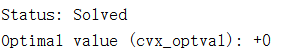# How to get the optimal value(cvx_optval)?

I want to solve the feasibility problem, and I will judge the feasibility of the problem according to the optimal value obtained by CVX. The optimal value(cvx_optval) is zero means the problem is feasible(as shown in illustration). But I don’t know how to get the optimal value(cvx_optval). Is there some matlab’s command to do that?Type at the command line
`cvx_optval`

Or save it to a MATLAB variable:
`cvx_optval_saved_value = cvx_optval;`